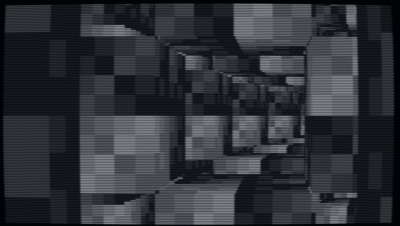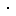# pouët.net

Exotic by Desire [web]
[nfo]platform : type : release date : october 2020
•20
•1
•0
popularity : 48%
48%
•0.95
alltime top: #14185
added on the 2020-10-08 23:10:00 by HellMood## popularity helper

increase the popularity of this prod by spreading this URL:

sweet, and for tiny computer :)
I like this tunnel
Cool :)
Nice one!
yes!
tic80 needs an own category just like picco8!
thumb fading in. and out. and in. and ...
Platform thumb

p.s. shaved off six chars
Code:`t=93 function TIC()for c=0,47 do r=math.abs(31-(t//3&63))poke(16320+c,c*r/6)end t=t+1 for x=-120,120 do for y=-68,68 do for d=-10,-99,-1 do h=((d*d+d*x~(y*d))//256+4)&(d-t)if h&8>0then pix(x+120,y+68,(h-7+((x~y)&1)))break end end end end end`
down to 237
Code:`t=93q=68s=120 function TIC()for c=0,47 do r=math.abs(31-(t//3&63))poke(16320+c,c*r/6)end t=t+1 for x=-s,s do for y=-q,q do for d=-10,-99,-1 do h=(d*d+d*x~(y*d))//256+4&(d-t)if h&8>0then pix(x+s,y+q,h-7+((x~y)&1))break end end end end end`
just a bunch of boxes moving :D
moar tic80s
Quote:
bunch of boxes moving

:]
:) - Great to see new things
So this is what it looks like when hellmood codes a prod in a reactive hissy fit
Very cool! And with source! ;)
just a bunch of cool boxes moving :D
Nice tunnel in small filesize on TIC-80
@hellmood how many additional bytes to make it rotate?
for the music
Very cool! I thought I was watching an MS-DOS intro.
Awesome.